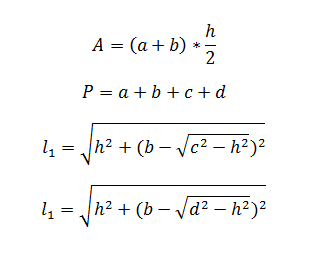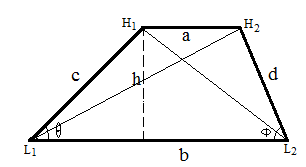# Trapezoid Calculator

This CalcTown calculator calculates area, perimeter and diagonal length of a trapezoid.

#### Result

m
m
m
mWhere,

a, b = parallel sides of the trapezoid

c,d = non-parallel sides of the trapezoid

h = altitude of the trapezoid

A = area of the trapezoid

P = perimeter of trapezoid

l1, l2 = diagonal lengths of the trapezoid